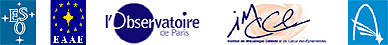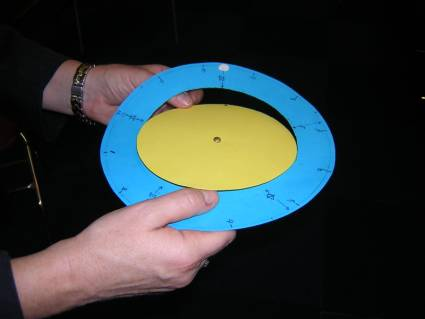# Why does the Transit of Venus occur rarely... and with a special rhythm? 

Level: Basic for understanding other concepts

### Objectives

• To understand why transits of Venus take place twice in 8 years (as in 2004 and 2012) and then only after an interval of about 120 years.
• To construct a model to understand the relative positions of Venus, the Earth and the Sun.

### Assumptions

We assume that:

• Venus and the Earth have circular orbits
• Venus and the Earth are coplanar (only in some cases)
• The sidereal period of Venus is an integer number of 7 months so Venus' orbit is represented by 7 intervals on the model. In reality, Venus' sidereal period is nearly 7.5 months so one extra interval has to be added to the Earth's motion each time Venus completes two revolutions.

### Background

Students need background information about Venus'cycle.
Astronomical contents:

### Material needed

• Blue and yellow cardboard
• A pair of scissors
• A paper fastener

### Resources

• Cardboard model: the motion of Venus
• Human model: the cycle of Venus
• Human model: the Venus pentagram on the zodiac

## Introduction

This series of activities develops a simple model for students to use in a variety of experiments related to the motion of Venus. First of all, students create a flat model to become familiar with the cycle of Venus. Later the model is modified in order to show that Venus' orbit is inclined relative to Earth's orbit, so transits take place only during those rare instances when Venus slips directly between the Earth and the Sun, twice in eight years (as in 2004 and 2012) and then only after an interval of about 120 years (in pairs, about once per century).A cardboard model to explain the movement of Venus

## The model: The motion of Venus

### Construction

1. Make a circle 20 cm in diameter (the Earth's orbit) on stiff blue paper that represents a plane in the sky, fixed with respect to the stars.
2. Cut out of stiff yellow paper a circle 14 cm in diameter (the orbit of Venus) on the same scale as the orbit of the Earth
3. Mark a point (Venus) on its orbit.
4. Mark the Sun at the very centre of the model
5. Indicate on the external border of the blue disc, at twelve regular intervals, the numbers from 0 to 11 (the start of Earth's months).
6. Indicate on an inner circle of the blue disc, at regular distances, seven points (the months of Venus' orbit).
7. Indicate on the blue disc, at regular distances, the positions from I to V (the conjunctions)
8. Place the yellow circle on top of the blue paper and push a paper fastener through the centre
9. Put a small ball of Plasticine (the Earth) on the Earth's orbit.

Instead of following the instructions from point 1 to 7, students can create the model from a master copy.

### Using the model

Now you have to follow the instructions step by step!

1. Start with Venus and the Earth both at the zero positions of their orbits. Venus is in the same direction as the Sun (conjunction) and is invisible from the Earth. The Earth and Venus move in their orbits, both anticlockwise, so this position doesn't last long. Venus needs 225 days (nearly 7 months) to turn once around the Sun, the Earth 365 days (12 months). These times are called the sidereal periods of Venus and the Earth. When Venus is exactly between Earth and Sun, as seen from the Earth, it will cross the Sun's disc. (Verify that Venus moves over the Sun's disc from left to right.)

2. Now move Venus and the Earth together, month by month, but be careful to make an adjustment to the model. We assumed Venus' sidereal period was seven months in order to simplify the counting, so Venus' orbit is represented by seven intervals on the model. As the actual sidereal period of Venus is nearly 7.5 months, Venus returns to the same point after two revolutions lasting nearly 15 months. One extra interval has also to be added to the Earth's motion. Where is the Earth when Venus has made one revolution around the Sun starting from position 0? ( solution 1) Did the Earth and Venus come into conjunction again during this time? ( solution 2)

3. Where is the Earth when Venus has completed its second revolution around the Sun? ( solution 3 Did a conjunction happen during this period? ( solution 4) How much time has passed on the Earth? ( solution 5)

4. Now we start a third revolution of Venus. Move Venus and the Earth together as regularly as possible. Verify that Venus and the Earth come into conjunction at the position indicated by I. How much time has passed (estimate years and months) after the first conjunction? ( solution 6)

5. Verify that after another synodic period, Earth and Venus are in position II. How many years have passed from the start at 0? ( solution 7) How many revolutions has Venus made in this time? ( solution 8)

6. Verify that the next two conjunctions are in the positions III and IV.

7. The fifth conjunction V from the beginning is again at point 0. Verify that eight years have now passed from the start.
• How many revolutions has the Earth made in this time? ( solution 9)
• How many revolutions has Venus made in this time? ( solution 10)
8. When the conditions are exactly like this, there should be five transits of Venus in eight years.

9. But why can we see only two transits in eight years?
It is because the model doesn't represent reality exactly. The orbits of Venus and the Earth are actually not exactly coplanar.
10. To make this situation clear in the model, carefully cut along the dotted half inner circle of the blue disc and fold it downwards along the black line (to do it, first remove the plane of Venus and then replace it again). The plane of the orbit of the Earth is called the Ecliptic. The line you folded along is called the nodal line; it is the intersection with the orbit of Venus. The angle between the orbit of Venus and the ecliptic is only about three degrees. In the model we use a bigger angle (about 20Âº-30Âº) to make things clearer.11. Place the Earth and Venus again in position 0. There is a conjunction and also a transit. Now place the Earth and Venus in positions I, II, III and IV. As you can see, there is a conjunction but not a transit: seen from the Earth, Venus is above or below the Sun-Earth plane (the Ecliptic). Only when the Earth and Venus arrive at position V is there again a transit, after eight years!

But why can we see a transit again only after about 120 years?
It is because the synodic period is not exactly 584 days, but 583.92 days. Five times 584 days is exactly eight years, but five times 583.92 days is somewhat less then eight years. So the conjunction doesn't take place exactly at the nodal line, but a bit before. Because the difference is not very great, eight years after the first transit Venus may still cross the Sun's disc, however along another line. The difference becomes greater and greater, so after another eight years the conjunction takes place too far from the nodal line to cross the Sun. So we have to wait until the conjunction takes place again at the position of the nodal line - that is after about 120 years.Written by Leonarda Fucili and Rosa M. Ros (EAAE).